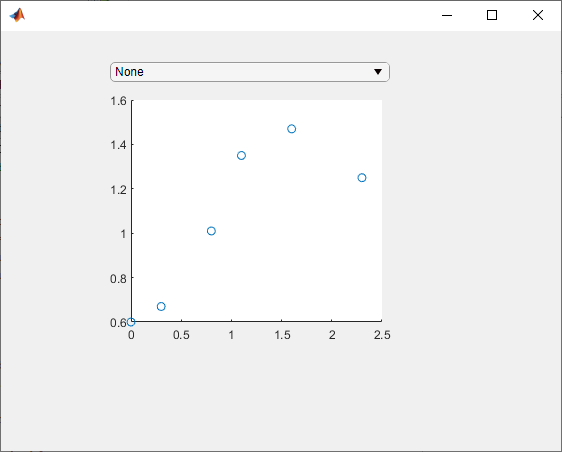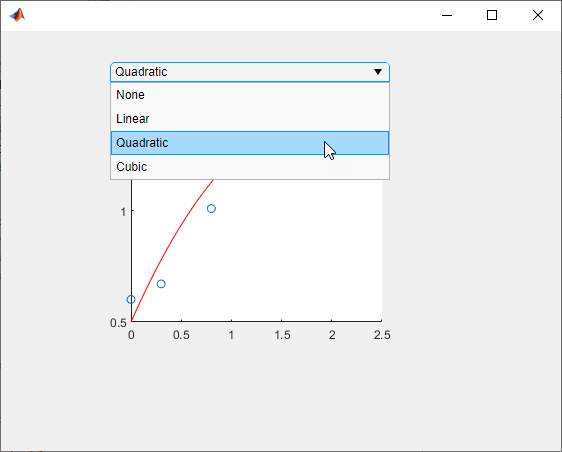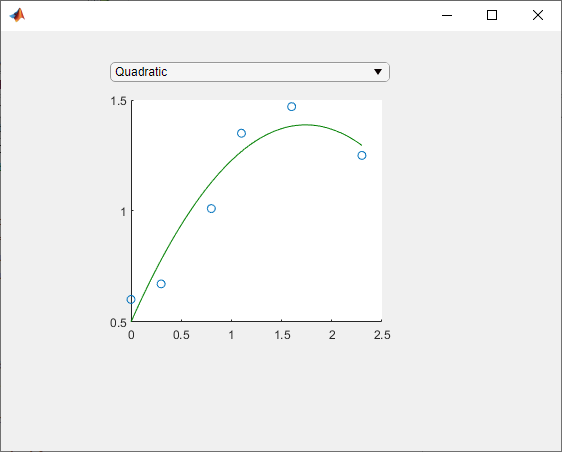Main Content

## Manage Properties of Custom UI Components

When you develop a custom UI component as a subclass of the `ComponentContainer` base class, you can use certain techniques to make your code more robust, efficient, and user-friendly. These techniques focus on how you define and manage the properties of your class. Use any that are helpful for the type of component you want to create and the user experience you want to provide.

• Initialize Property Values — Set the default state of the UI component in case your users call the implicit constructor without any input arguments.

• Validate Property Values — Ensure that the values are valid before using them.

• Customize the Property Display — Provide a customized list of properties in the Command Window when a user references the UI component object without a semicolon.

• Optimize the update Method — Improve the performance of the `update` method when only a subset of your properties are used in a time-consuming calculation.

For an example of these techniques, see Example: Optimized Polynomial Fit UI Component with Customized Property Display.

In addition, there are certain considerations and limitations to keep in mind if you want to use your custom UI component in App Designer, or share your component with users who develop apps in App Designer. These considerations are listed on a separate page, in Configure Custom UI Components for App Designer.

### Initialize Property Values

Assign default values for all of the public properties of your class. This allows MATLAB to create a valid UI component even if the user omits some name-value arguments when they call the constructor method.

For UI components that contain a chart and have properties that store coordinate data, set the initial values to `NaN` values or empty arrays so that the default chart is empty when the user does not specify the coordinates.

### Validate Property Values

Before your code uses property values, confirm that they have the correct size and class. For example, this property block validates the size and class of three properties.

```properties LineColor {validateattributes(LineColor,{'double'}, ... {'<=',1,'>=',0,'size',[1 3]})} = [1 0 0] XData (1,:) double = NaN YData (1,:) double = NaN end```

`LineColor` must be a 1-by-3 array of class `double`, where each value is in the range `[0,1]`. Both `XData` and `YData` must be row vectors of class `double`.

You can also validate properties that store the underlying component objects in your UI component. To do this, you need to know the correct class name for each object. To determine the class name of an object, call the corresponding UI component function at the command line, and then call the `class` function to get the class name. For example, if you plan to create a drop-down component in your `setup` method, call the `uidropdown` function at the command line with an output argument. Then, pass the output to the `class` function to get its class name.

```dd = uidropdown; class(d)```
```ans = 'matlab.ui.control.DropDown'```

Use the output of the `class` function to validate the class for the corresponding property in your class. Specify the class after the property name. For example, the following property stores a `DropDown` object and validates its class.

```properties (Access = private, Transient, NonCopyable) DropDown matlab.ui.control.DropDown end```

Occasionally, you might want to define a property that can store different shapes and classes of values. For example, if you define a property that can store a character vector, cell array of character vectors, or string array, omit the size and class validation or use a custom property validation method. For more information about validating properties, see Validate Property Values.

### Customize the Property Display

One of the benefits of defining your UI component as a subclass of the `ComponentContainer` base class is that it also inherits from the `matlab.mixin.CustomDisplay` class. This lets you customize the list of properties MATLAB® displays in the Command Window when you reference the UI component without a semicolon. To customize the property display, overload the `getPropertyGroups` method. Within that method, you can customize which properties are listed and the order of the list. For example, consider a `FitPlot` class that has the following public properties.

```properties LineColor {validateattributes(LineColor,{'double'}, ... {'<=',1,'>=',0,'size',[1 3]})} = [1 0 0] XData (1,:) double = NaN YData (1,:) double = NaN end```

The following `getPropertyGroups` method specifies the scalar object property list as `XData`, `YData`, and `LineColor`.

```function propgrp = getPropertyGroups(obj) if ~isscalar(obj) % List for array of objects propgrp = getPropertyGroups@matlab.mixin.CustomDisplay(obj); else % List for scalar object propList = {'XData','YData','LineColor'}; propgrp = matlab.mixin.util.PropertyGroup(propList); end end ```

When the user references an instance of this UI component without a semicolon, MATLAB displays the customized list.

`p = FitPlot`
```p = FitPlot with properties: XData: NaN YData: NaN LineColor: [1 0 0]```

For more information about customizing the property display, see Customize Property Display.

### Optimize the `update` Method

In most cases, the `update` method of your class reconfigures all the relevant aspects of your UI component that depend on the public properties. Sometimes, the reconfiguration involves an expensive calculation that is time consuming. If the calculation involves only a subset of the properties, you can design your class to execute that code only when it is necessary.

One way to optimize the `update` method is to add these elements to your class:

• A private property called `ExpensivePropChanged` that accepts a `logical` value. This property indicates whether any of the properties used in the expensive calculation have changed.

• A `set` method for each property involved in the expensive calculation. Within each `set` method, set the `ExpensivePropChanged` property to `true`.

• A protected method called `doExpensiveCalculation` that performs the expensive calculation.

• A conditional statement in the `update` method that checks the value of `ExpensivePropChanged`. If the value is `true`, execute `doExpensiveCalculation`.

The following code provides a template for this design.

```classdef OptimizedUIComponent < matlab.ui.componentcontainer.ComponentContainer properties Prop1 Prop2 end properties(Access=private,Transient,NonCopyable) ExpensivePropChanged (1,1) logical = true end methods(Access = protected) function setup(obj) % Configure UI component % ... end function update( obj ) % Perform expensive computation if needed if obj.ExpensivePropChanged doExpensiveCalculation(obj); obj.ExpensivePropChanged = false; end % Update other aspects of UI component % ... end function doExpensiveCalculation(obj) % Expensive code % ... end end methods function set.Prop2(obj,val) obj.Prop2 = val; obj.ExpensivePropChanged = true; end end end```

In this case, `Prop2` is involved in the expensive calculation. The `set.Prop2` method sets the value of `Prop2`, and then it sets `ExpensivePropChanged` to `true`. The next time the `update` method runs, it calls `doExpensiveCalculation` only if `ExpensivePropChanged` is `true`. Then, the `update` method continues to update other aspects of the UI component.

### Example: Optimized Polynomial Fit UI Component with Customized Property Display

This example defines a `FitPlot` class for interactively displaying best fit polynomials, and uses all four of these best practices. The properties defined in the properties block have default values and use size and class validation. The `getPropertyGroups` method defines a custom order for the property display. The `changeFit` method performs the potentially expensive polynomial fit calculation, and the `update` method executes `changeFit` only if the plotted data changed.

To define this class, save the `FitPlot` class definition to a file named `FitPlot.m` in a folder that is on the MATLAB path.

```classdef FitPlot < matlab.ui.componentcontainer.ComponentContainer % Choose a fit method for your plotted data properties LineColor {validateattributes(LineColor,{'double'}, ... {'<=',1,'>=',0,'size',[1 3]})} = [1 0 0] XData (1,:) double = NaN YData (1,:) double = NaN end properties (Access = private, Transient, NonCopyable) DropDown matlab.ui.control.DropDown Axes matlab.ui.control.UIAxes GridLayout matlab.ui.container.GridLayout DataLine (1,1) matlab.graphics.chart.primitive.Line FitLine (1,1) matlab.graphics.chart.primitive.Line FitXData (1,:) double FitYData (1,:) double ExpensivePropChanged (1,1) logical = true end methods (Access=protected) function setup(obj) % Set the initial position of this component obj.Position = [100 100 300 300]; % Create the grid layout, drop-down, and axes obj.GridLayout = uigridlayout(obj,[2,1], ... 'RowHeight',{20,'1x'},... 'ColumnWidth',{'1x'}); obj.DropDown = uidropdown(obj.GridLayout, ... 'Items',{'None','Linear','Quadratic','Cubic'}, ... 'ValueChangedFcn',@(s,e) changeFit(obj)); obj.Axes = uiaxes(obj.GridLayout); % Create the line objects obj.DataLine = plot(obj.Axes,NaN,NaN,'o'); hold(obj.Axes,'on'); obj.FitLine = plot(obj.Axes,NaN,NaN); hold(obj.Axes,'off'); end function update(obj) % Update data points obj.DataLine.XData = obj.XData; obj.DataLine.YData = obj.YData; % Do an expensive operation if obj.ExpensivePropChanged obj.changeFit(); obj.ExpensivePropChanged = false; end % Update the fit line obj.FitLine.Color = obj.LineColor; obj.FitLine.XData = obj.FitXData; obj.FitLine.YData = obj.FitYData; end function changeFit(obj) % Calculate the fit line based on the drop-down value if strcmp(obj.DropDown.Value,'None') obj.FitXData = NaN; obj.FitYData = NaN; else switch obj.DropDown.Value case 'Linear' f = polyfit(obj.XData,obj.YData,1); case 'Quadratic' f = polyfit(obj.XData,obj.YData,2); case 'Cubic' f = polyfit(obj.XData,obj.YData,3); end obj.FitXData = linspace(min(obj.XData),max(obj.XData)); obj.FitYData = polyval(f,obj.FitXData); end end function propgrp = getPropertyGroups(obj) if ~isscalar(obj) % List for array of objects propgrp = getPropertyGroups@matlab.mixin.CustomDisplay(obj); else % List for scalar object propList = {'XData','YData','LineColor'}; propgrp = matlab.mixin.util.PropertyGroup(propList); end end end methods function set.XData(obj,val) obj.XData = val; obj.ExpensivePropChanged = true; end function set.YData(obj,val) obj.YData = val; obj.ExpensivePropChanged = true; end end end```

Define some sample data and use it to create an instance of `FitPlot`.

```x = [0 0.3 0.8 1.1 1.6 2.3]; y = [0.6 0.67 1.01 1.35 1.47 1.25]; p = FitPlot('XData',x,'YData',y)```
```ans = FitPlot with properties: XData: [1×43 double] YData: [1×43 double] LineColor: [1 0 0]```Use the drop-down to display the quadratic best fit curve.Set the `LineColor` property to change the color of the best fit curve to green.

`p.LineColor = [0 0.5 0];`Download ebook